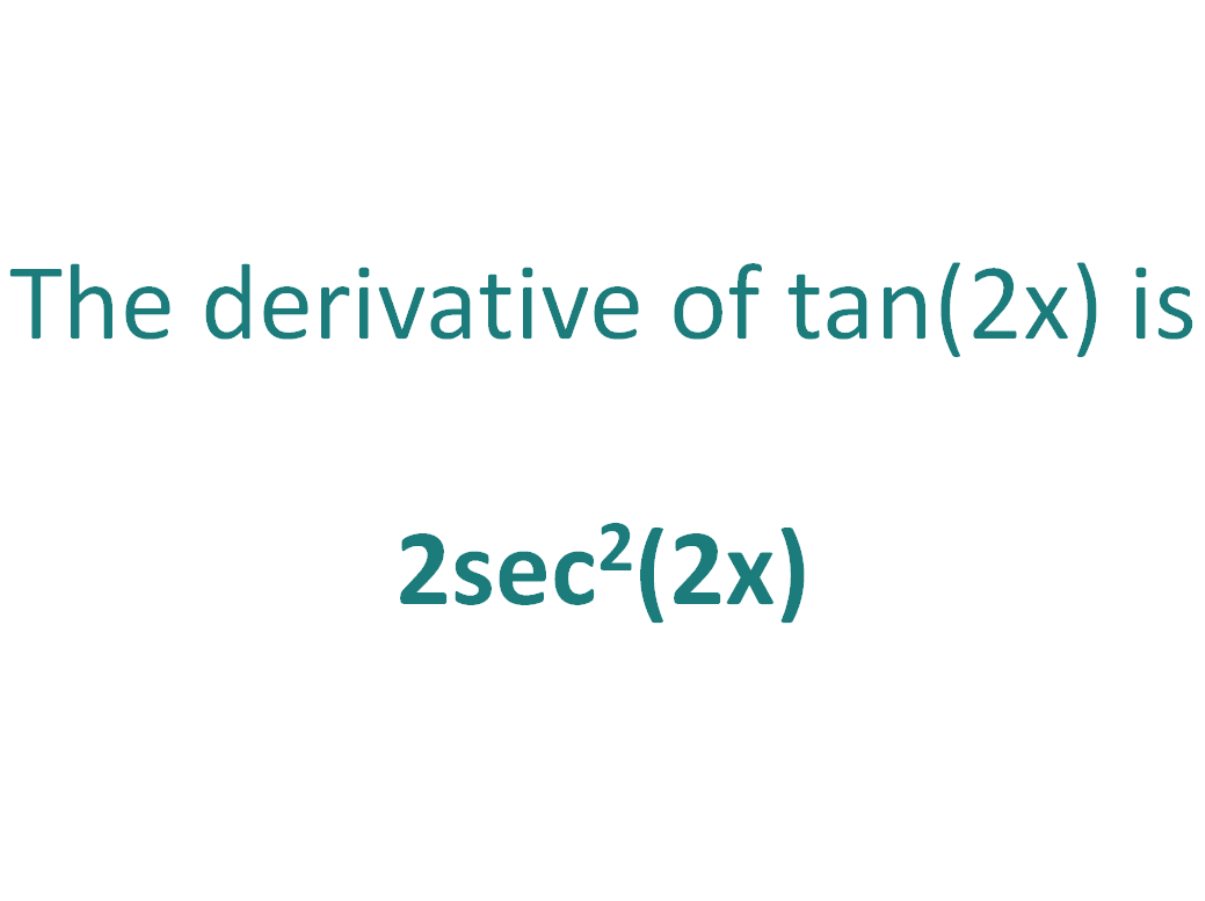# The Derivative of tan(2x)The derivative of tan(2x) is 2sec2(2x)

## How to calculate the derivative of tan(2x)

Note that in this post we will be looking at differentiating tan(2x) which is not the same as differentiating tan2(x). Here is our post dealing with how to differentiate tan^2(x).

The chain rule is useful for finding the derivative of a function which could have been differentiated had it been in x, but it is in the form of another expression which could also be differentiated if it stood on its own.

In this case:

• We know how to differentiate tan(x) (the answer is sec2(x))
• We know how to differentiate 2x (the answer is 2)

This means the chain rule will allow us to differentiate the expression tan(2x).

#### Using the chain rule to find the derivative of tan(2x)

To perform the differentiation tan(2x), the chain rule says we must differentiate the expression as if it were just in terms of x as long as we then multiply that result by the derivative of what the expression is actually in terms of (in this case the derivative of 2x).

Let’s call the function in the argument of tan, g(x), which means the function is in the form of tan(x), except it does not have x as the angle, instead it has another function of x (2x) as the angle

If:

g(x) = 2x

It follows that:

tan(2x) = tan(g(x))

So if the function f(x) = tan(x) and the function g(x) = 2x, then the function tan(2x) can be written as a composite function.

f(x) = tan(x)

f(g(x)) = tan(g(x)) (but g(x) = 2x))

f(g(x)) = tan(2x)

Let’s define this composite function as F(x):

F(x) = f(g(x)) = tan(2x)

We can find the derivative of tan(2x) (F'(x)) by making use of the chain rule.

The Chain Rule:
For two differentiable functions f(x) and g(x)

If F(x) = f(g(x))

Then the derivative of F(x) is F'(x) = f’(g(x)).g’(x)

Now we can just plug f(x) and g(x) into the chain rule. But before we do that, just a quick recap on the derivative of the tan function.

The derivative of tan(x) with respect to x is sec2(x)
The derivative of tan(z) with respect to z is sec2(z)

In a similar way, the derivative of tan(2x) with respect to 2x is sec2(2x).

We will use this fact as part of the chain rule to find the derivative of tan(2x) with respect to x.

How to find the derivative of tan(2x) using the Chain Rule:

Using the chain rule, the derivative of tan(2x) is 2sec2(2x)

Finally, just a note on syntax and notation: tan(2x) is sometimes written in the forms below (with the derivative as per the calculation above). Just be aware that not all of the forms below are mathematically correct.

## The Second Derivative Of tan(2x)

To calculate the second derivative of a function, you just differentiate the first derivative.

From above, we found that the first derivative of tan(2x) = 2sec2(2x). So to find the second derivative of tan(2x), we just need to differentiate 2sec2(2x).

We can use the chain rule to find the derivative of 2sec2(2x) (bearing in mind that the derivative of sec^2(x) is 2sec2(x)tan(x)) and it gives us a result of 8sec2(2x)tan(2x)

The second derivative of tan(2x) is 8sec2(2x)tan(2x)